Next: Which are the Up: Famous Problems in Mathematics Previous: The Four Colour Theorem

# The Trisection of an Angle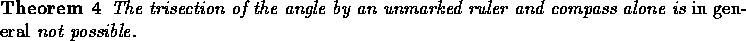This problem, together with Doubling the Cube, Constructing the regular Heptagon and Squaring the Circle were posed by the Greeks in antiquity, and remained open until modern times.

The solution to all of them is rather inelegant from a geometric perspective. No geometric proof has been offered [check?], however, a very clever solution was found using fairly basic results from extension fields and modern algebra.

It turns out that trisecting the angle is equivalent to solving a cubic equation. Constructions with ruler and compass may only compute the solution of a limited set of such equations, even when restricted to integer coefficients. In particular, the equation for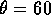degrees cannot be solved by ruler and compass and thus the trisection of the angle is not possible.

It is possible to trisect an angle using a compass and a ruler marked in 2 places.

Suppose X is a point on the unit circle such that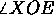is the angle we would like to ``trisect''. Draw a line AX through a point A on the x-axis such that |AB| = 1 (which is the same as the radius of the circle), where B is the intersection-point of the line AX with the circle.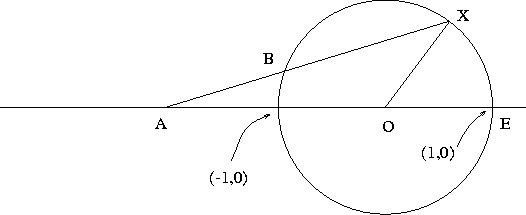Figure 7.1: Trisection of the Angle with a marked ruler

Letbe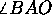. Then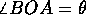, and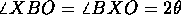Since the sum of the internal angles of a triangle equals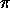radians (180 degrees) we have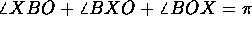, implying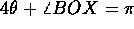. Also, we have that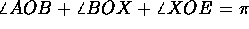, implying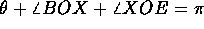. Since both quantities are equal towe obtain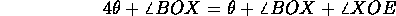From which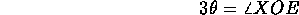follows. QED.

Alex Lopez-Ortiz
Mon Feb 23 16:26:48 EST 1998